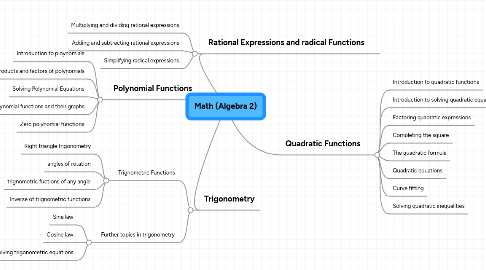# Math (Algebra 2)

Get Started. It's FreeMath (Algebra 2)## 3. Trigonometry

### 3.1. Trignometric Functions

3.1.1. Right triangle trigonometry

3.1.2. angles of rotation

3.1.3. trignometric fuctions of any angle

3.1.4. Inverse of trignometric functions

### 3.2. Further topics in trigonometry

3.2.1. Sine law

3.2.2. Cosine law

3.2.3. Solving trigonometric equations